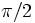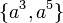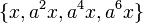# Element structure of dihedral group:D16

View element structure of particular groups | View other specific information about dihedral group:D16

This article describes the structure of elements of the dihedral group:D16, which is given by the following presentation:$\langle a,x \mid a^8 = x^2 = e, xax = a^{-1} \rangle$

Here,$e$ denotes the identity element. Every element is of the form$a^k, 0 \le k \le 7$ or$a^kx, 0 \le k \le 7$.

## Elements

Below, we list all the elements, also giving the interpretation of each element under the geometric description of the dihedral group as the symmetries of a 8-gon, and for the corresponding permutation representation (see D16 in S8). Note that for different conventions, one can obtain somewhat different correspondences, so this may not match up with other correspondences elsewhere. Note that the descriptions below assume the left action convention for functions and the corresponding convention for composition, and hence some of the entries may become different if you adopt the right action convention.:

Element in terms of$a$ and$x$ Geometric description of action on regular octagon Permutation on vertices Order of the element$e$ does nothing, i.e., leaves the 8-gon fixed$()$ 1$a$ counter-clockwise rotation by$\pi/4$ (i.e.,$45\,^\circ$)$(1,2,3,4,5,6,7,8)$ 8$a^2$ counter-clockwise rotation by$\pi/2$ (i.e.,$90\,^\circ$)$(1,3,5,7)(2,4,6,8)$ 4$a^3$ counter-clockwise rotation by$3\pi/4$ (i.e.,$135\,^\circ$)$(1,4,7,2,5,8,3,6)$ 8$a^4$ rotation by$\pi$ (i.e.,$180\,^\circ$), or half turn$(1,5)(2,6)(3,7)(4,8)$ 2$a^5$ counter-clockwise rotation by$5\pi/4$ (i.e.,$225\,^\circ$) or$3\pi/4$ clock-wise$(1,6,3,8,5,2,7,4)$ 8$a^6$ counter-clockwise rotation by$3\pi/2$ (i.e.,$270\,^\circ$) or$\pi/2$ clock-wise$(1,7,5,3)(2,8,6,4)$ 4$a^7$ counter-clockwise rotation by$7\pi/4$ (i.e.,$315\,^\circ$) or$\pi/4$ clock-wise$(1,8,7,6,5,4,3,2)$ 8$x$ 2$ax$ 2$a^2x$ 2$a^3x$ 2$a^4x$ 2$a^5x$ 2$a^6x$ 2$a^7x$ 2

## Conjugacy class structure

FACTS TO CHECK AGAINST FOR CONJUGACY CLASS SIZES AND STRUCTURE:
Divisibility facts: size of conjugacy class divides order of group | size of conjugacy class divides index of center | size of conjugacy class equals index of centralizer
Bounding facts: size of conjugacy class is bounded by order of derived subgroup
Counting facts: number of conjugacy classes equals number of irreducible representations | class equation of a group
Conjugacy class Size of conjugacy class Order of elements in conjugacy class Centralizer of first element of class$\! \{ e \}$ 1 1 whole group$\! \{ a^4 \}$ 1 2 whole group$\! \{ a^2, a^6 \}$ 2 4$\langle a \rangle$ -- a cyclic subgroup of order$8$$\! \{ a, a^7 \}$ 2 8$\langle a \rangle$ -- a cyclic subgroup of order$8$$\! \{ a^3, a^5 \}$ 2 8$\langle a \rangle$ -- a cyclic subgroup of order$8$$\! \{ x, a^2x, a^4x, a^6x \}$ 4 2$\! \{ e, a^4, x, a^4x \}$$\! \{ ax, a^3x, a^5x, a^7x \}$ 4 2$\! \{ e, a^4, ax, a^5x \}$

## Classes up to automorphisms

Equivalence class under automorphisms Order of elements Size of equivalence class Number of conjugacy classes in it Size of each conjugacy class$\! \{ e \}$ 1 1 1 1$\! \{ a^4 \}$ 2 1 1 1$\! \{ a^2,a^6 \}$ 4 2 1 2$\! \{ a, a^3, a^5, a^7 \}$ 8 4 2 2$\! \{ x, ax, a^2x, a^3x, a^4x, a^5x, a^6x, a^7x \}$ 2 8 2 4

## Order and power information

### Order statistics

Number Elements of order exactly that number Number of such elements Number of conjugacy classes of such elements Number of elements whose order divides that number Number of conjugacy classes whose element order divides that number
1$\! \{ e \}$ 1 1 1 1
2$\! \{ a^4, x, ax, a^2x, a^3x, a^4x, a^5x, a^6x, a^7x \}$ 9 3 10 4
4$\! \{ a^2, a^6 \}$ 2 1 12 5
8$\! \{ a, a^3, a^5, a^7 \}$ 4 2 16 7

### Power statistics

Number$d$$d^{th}$ powers that are not$k^{th}$ powers for any larger divisor$k$ of the group order Number of such elements Number of conjugacy classes of such elements Number of$d^{th}$ powers Number of conjugacy classes of$d^{th}$ powers
1$\! \{ a, a^3, a^5, a^7, x, ax, a^2x, a^3x, a^4x, a^5x, a^6x, a^7x \}$ 12 4 16 7
2$\! \{ a^2, a^6 \}$ 2 1 4 3
4$\! \{ a^4 \}$ 1 1 2 2
8 -- 0 0 1 1
16$\! \{ e \}$ 1 1 1 1